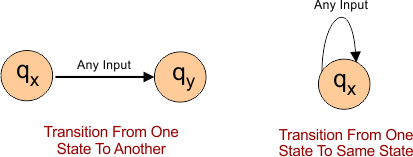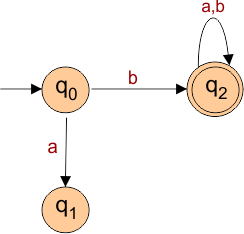Select Page

# Transition In Automata

Moving From one state to another or in same state with the help of arrow, by using an input alphabet is called transition in Automata. Transition happens with help of an input symbol and some rules.

### Notation of Transition

Notation of transition is given below### Example:

DFA with ∑ = {0, 1} accepts all strings starting with 1.

Solution:• In above finite automata transition graph, the machine initially is in initial state (q0) then on receiving input “b” the machine changes its state to q2.
• From q0 on receiving “a”, the machine changes its state to q1 from q0, which is the dead state.
• From q2 on receiving input “a”, “b” the machine changes its state to q2, which is the final state.
• The possible input strings that can be generated are ba, bb, bba, bab, bbb……., that means all string starts with “b”.
• In simple words, above machine can accept all strings which are starting with “b”.

## Transition Table

Representation of the transition function in Tabular form is called transition table. Columns in Transition table are explained below

• First Column for all possible states.
• Rest all Columns for fix for each corresponding input symbols. It is also called next state after transition for specific corresponding input symbols.

Note: The initial state is denoted by an arrow with no source and final state is denoted by a star.

### ExampleSolution:

Transition table of above DFA is given below

 Present State Next state for Input “a” Next State of Input “b” →q0 q1 q2 q1 q0 q2 *q2 q2 q2

Explanation:

• In the above transition table, the first column shows all the states and rest of the columns shows next state for each input.

The first row of the transition table Shows

• The current state is q0, on input ”a” the next state will be q1 and on input “b” the next state will be q2.

The Second row of the transition table Shows

• The current state is q1, on input “a”, the next state will be q0, and on input “b” the next state will be q2.

The Third row of the transition table Shows

• The current state is q2 on input “a”, the next state will be q2, and on “b” input the next state will be q2.

Help Other’s By Sharing…